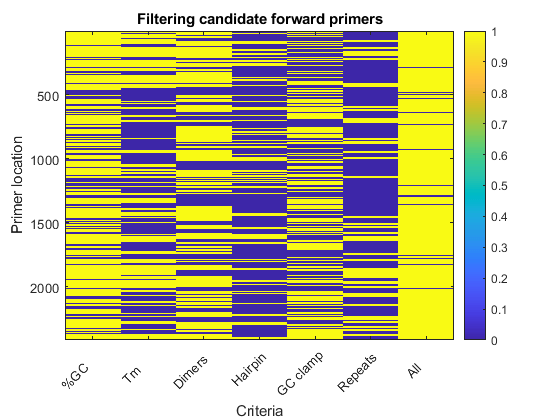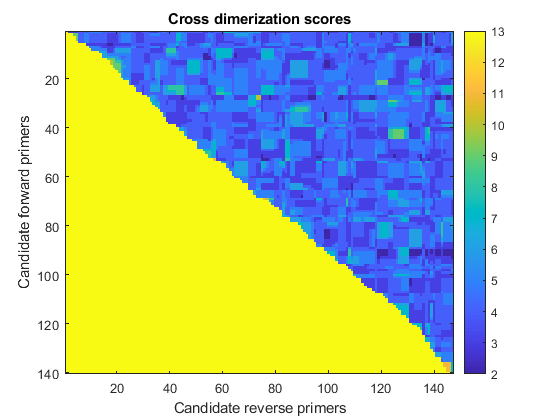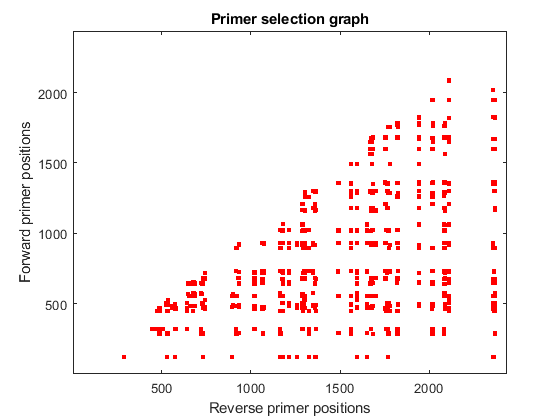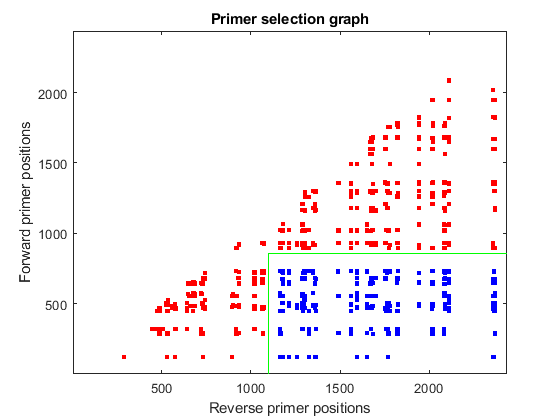Documentation

## Exploring Primer Design

This example shows how to use the Bioinformatics Toolbox™ to find potential primers that can be used for automated DNA sequencing.

### Introduction

Primer design for PCR can be a daunting task. A good primer should usually meet the following criteria:

• Length is 18-30 bases.

• Melting temperature is 50-60 degrees Celsius.

• GC content is between 45% and 55%.

• Does not form stable hairpins.

• Does not self dimerize.

• Does not cross dimerize with other primers in the reaction.

• Has a GC clamp at the 3' end of the primer.

This example uses MATLAB® and Bioinformatics Toolbox to find PCR primers for the human hexosaminidase gene.

First load the hexosaminidase nucleotide sequence from the provided MAT-file `hexosaminidase.mat`. The DNA sequence that you want to find primers for is in the `Sequence` field of the loaded structure.

```load('hexosaminidase.mat','humanHEXA') sequence = humanHEXA.Sequence; ```

You can also use the `getgenbank` function to retrieve the sequence information from the NCBI data repository and load it into MATLAB. The NCBI reference sequence for HEXA has accession number NM_000520.

```humanHEXA = getgenbank('NM_000520'); ```

### Calculating Properties of an Oligonucleotide

The `oligoprop` function is a useful tool to get properties of oligonucleotide DNA sequences. This function calculates the GC content, molecular weight, melting temperature, and secondary structure information. `oligoprop` returns a structure that has fields with the associated information. Use the `help` command to see what other properties `oligoprop` returns.

```nt = oligoprop('AAGCTCAAAAACGCGCGGTATTCGACTGGCGTGATCTATTTTATGCT') ```
```nt = struct with fields: GC: 44.6809 GCdelta: 0 Hairpins: [3x47 char] Dimers: [9x47 char] MolWeight: 1.4468e+04 MolWeightdelta: 0 Tm: [68.9128 79.7752 85.3393 69.6497 68.2474 75.8931] Tmdelta: [0 0 0 0 0 0] Thermo: [4x3 double] Thermodelta: [4x3 double] ```

### Finding All Potential Forward Primers

The goal is to create a list of all potential forward primers of length 20. You can do this either by iterating over the entire sequence and taking subsequences at every possible position or by using a matrix of indices. The following example shows how you can set a matrix of indices and then use it to create all possible forward subsequences of length 20, in this case N-19 subsequences where N is the length of the target hexosaminidase sequence. Then you can use the `oligoprop` function to get properties for each of the potential primers in the list.

```N = length(sequence) % length of the target sequence M = 20 % desired primer length index = repmat((0:N-M)',1,M)+repmat(1:M,N-M+1,1); fwdprimerlist = sequence(index); for i = N-19:-1:1 % reverse order to pre-allocate structure fwdprimerprops(i) = oligoprop(fwdprimerlist(i,:)); end ```
```N = 2437 M = 20 ```

### Finding All Potential Reverse Primers

After you have all potential forward primers, you can search for reverse primers in a similar fashion. Reverse primers are found on the complementary strand. To obtain the complementary strand use the `seqcomplement` function.

```comp_sequence = seqcomplement(sequence); revprimerlist = seqreverse(comp_sequence(index)); for i = N-19:-1:1 % reverse order to preallocate structure revprimerprops(i) = oligoprop(revprimerlist(i,:)); end ```

### Filtering Primers Based on GC Content

The GC content information for the primers is in a structure with the field GC. To eliminate all potential primers that do not meet the criteria stated above (a GC content of 45% to 55%), you can make a logical indexing vector that indicates which primers have GC content outside the acceptable range. Extract the GC field from the structure and convert it to a numeric vector.

```fwdgc = [fwdprimerprops.GC]'; revgc = [revprimerprops.GC]'; bad_fwdprimers_gc = fwdgc < 45 | fwdgc > 55; bad_revprimers_gc = revgc < 45 | revgc > 55; ```

### Filtering Primers Based on Their Melting Temperature

The melting temperature is significant when you are designing PCR protocols. Create another logical indexing vector to keep track of primers with bad melting temperatures. The melting temperatures from `oligoprop` are estimated in a variety of ways (basic, salt-adjusted, nearest-neighbor). The following example uses the nearest-neighbor estimates for melting temperatures with parameters established by SantaLucia, Jr. . These are stored in the fifth element of the field `Tm` returned by `oligoprop`. The other elements of this field represent other methods to estimate the melting temperature. You can also use the `mean` function to compute an average over all the estimates.

```fwdtm = cell2mat({fwdprimerprops.Tm}'); revtm = cell2mat({revprimerprops.Tm}'); bad_fwdprimers_tm = fwdtm(:,5) < 50 | fwdtm(:,5) > 60; bad_revprimers_tm = revtm(:,5) < 50 | revtm(:,5) > 60; ```

### Finding Primers With Self-Dimerization and Hairpin Formation

Self-dimerization and hairpin formation can prevent the primer from binding to the target sequence. As above, you can create logical indexing vectors to indicate whether the potential primers do or do not form self-dimers or hairpins.

```bad_fwdprimers_dimers = ~cellfun('isempty',{fwdprimerprops.Dimers}'); bad_fwdprimers_hairpin = ~cellfun('isempty',{fwdprimerprops.Hairpins}'); bad_revprimers_dimers = ~cellfun('isempty',{revprimerprops.Dimers}'); bad_revprimers_hairpin = ~cellfun('isempty',{revprimerprops.Hairpins}'); ```

### Finding Primers Without a GC Clamp

A strong base pairing at the 3' end of the primer is helpful. Find all the primers that do not end in a G or C. Remember that all the sequences in the lists are 5'->3'.

```bad_fwdprimers_clamp = lower(fwdprimerlist(:,end)) == 'a' | lower(fwdprimerlist(:,end)) == 't'; bad_revprimers_clamp = lower(revprimerlist(:,end)) == 'a' | lower(revprimerlist(:,end)) == 't'; ```

### Finding Primers With Nucleotide Repeats

Primers that have stretches of repeated nucleotides can give poor PCR results. These are sequences with low complexity. To eliminate primers with stretches of four or more repeated bases, use the function `regexp`.

```fwdrepeats = regexpi(cellstr(fwdprimerlist),'a{4,}|c{4,}|g{4,}|t{4,}','ONCE'); revrepeats = regexpi(cellstr(revprimerlist),'a{4,}|c{4,}|g{4,}|t{4,}','ONCE'); bad_fwdprimers_repeats = ~cellfun('isempty',fwdrepeats); bad_revprimers_repeats = ~cellfun('isempty',revrepeats); ```

### Find the Primers That Satisfy All the Criteria

The rows of the original list of subsequences correspond to the base number where each subsequence starts. You can use the logical indexing vectors collected so far and create a new list of primers that satisfy all the criteria discussed above. The figure shows how the forward primers have been filtered, where values equal to 1 indicates bad primers and values equal to 0 indicates good primers.

```bad_fwdprimers = [bad_fwdprimers_gc, bad_fwdprimers_tm,... bad_fwdprimers_dimers, bad_fwdprimers_hairpin,... bad_fwdprimers_clamp, bad_fwdprimers_repeats]; bad_revprimers = [bad_revprimers_gc, bad_revprimers_tm,... bad_revprimers_dimers, bad_revprimers_hairpin,... bad_revprimers_clamp, bad_revprimers_repeats]; good_fwdpos = find(all(~bad_fwdprimers,2)); good_fwdprimers = fwdprimerlist(good_fwdpos,:); good_fwdprop = fwdprimerprops(good_fwdpos); N_good_fwdprimers = numel(good_fwdprop) good_revpos = find(all(~bad_revprimers,2)); good_revprimers = revprimerlist(good_revpos,:); good_revprop = revprimerprops(good_revpos); N_good_revprimers = numel(good_revprop) figure imagesc([bad_fwdprimers any(bad_fwdprimers,2)]); title('Filtering candidate forward primers'); ylabel('Primer location'); xlabel('Criteria'); ax = gca; ax.XTickLabel = char({'%GC','Tm','Dimers','Hairpin','GC clamp','Repeats','All'}); ax.XTickLabelRotation = 45; colorbar ```
```N_good_fwdprimers = 140 N_good_revprimers = 147 ```### Checking For Cross Dimerization

Cross dimerization can occur between the forward and reverse primer if they have a significant amount of complementarity. The primers will not function properly if they dimerize with each other. To check for dimerization, align every forward primer against every reverse primer, using the `swalign` function, and keep the low-scoring pairs of primers. This information can be stored in a matrix with rows representing forward primers and columns representing reverse primers. This exhaustive calculation can be quite time-consuming. However, there is no point in performing this calculation on primer pairs where the reverse primer is upstream of the forward primer. Therefore, these primer pairs can be ignored. The image in the figure shows the pairwise scores before being thresholded, low scores (dark blue) represent primer pairs that do not dimerize.

```scr_mat = [-1,-1,-1,1;-1,-1,1,-1;-1,1,-1,-1;1,-1,-1,-1;]; scr = zeros(N_good_fwdprimers,N_good_revprimers); for i = 1:N_good_fwdprimers for j = 1:N_good_revprimers if good_fwdpos(i) < good_revpos(j) scr(i,j) = swalign(good_fwdprimers(i,:), good_revprimers(j,:), ... 'SCORINGMATRIX',scr_mat,'GAPOPEN',5,'ALPHA','NT'); else scr(i,j) = 13; % give a high score to ignore forward primers % that are after reverse primers end end end figure imagesc(scr) title('Cross dimerization scores') xlabel('Candidate reverse primers') ylabel('Candidate forward primers') colorbar ```Low scoring primer pairs are identified as logical one in an indicator matrix.

```pairedprimers = scr<=3; ```

### Visualizing Potential Pairs of Primers in the Sequence Domain

An alternative way to present this information is to look at all potential combinations of primers in the sequence domain. Each dot in the plot represents a possible combination between the forward and reverse primers after filtering out all those cases with potential cross dimerization.

```[f,r] = find(pairedprimers); figure plot(good_revpos(r),good_fwdpos(f),'r.','markersize',10) axis([1 N 1 N]) title('Primer selection graph') xlabel('Reverse primer positions') ylabel('Forward primer positions') ```### Selecting a Primer Pair to Amplify a Specific Region

You can use the information calculated so far to find the best primer pairs that allow amplification of the 220bp region from position 880 to 1100. First, you find all pairs that can cover the required region, taking into account the length of the primer. Then, you calculate the Euclidean distance of the actual positions to the desired ones, and re-order the list starting with the closest distance.

```pairs = find(good_fwdpos(f)<(880-M) & good_revpos(r)>1100); dist = (good_fwdpos(f(pairs))-(880-M)).^2 + (good_revpos(r(pairs))-(1100)).^2; [dist,h] = sort(dist); pairs = pairs(h); hold on plot(good_revpos(r(pairs)),good_fwdpos(f(pairs)),'b.','markersize',10) plot([1100 1100],[1 880-M],'g') plot([1100 N],[880-M 880-M],'g') ```### Retrieve Primer Pairs

Use the `sprintf` function to generate a report with the ten best pairs and associated information. These primer pairs can then be verified experimentally. These primers can also be 'BLASTed' using the `blastncbi` function to check specificity.

```Primers = sprintf('Fwd/Rev Primers Start End %%GC mT Length\n\n'); for i = 1:10 fwd = f(pairs(i)); rev = r(pairs(i)); Primers = sprintf('%s%-21s%-6d%-6d%-4.4g%-4.4g\n%-21s%-6d%-6d%-4.4g%-7.4g%-6d\n\n', ... Primers, good_fwdprimers(fwd,:),good_fwdpos(fwd),good_fwdpos(fwd)+M-1,good_fwdprop(fwd).GC,good_fwdprop(fwd).Tm(5), ... good_revprimers(rev,:),good_revpos(rev)+M-1,good_revpos(rev),good_revprop(rev).GC,good_revprop(rev).Tm(5), ... good_revpos(rev) - good_fwdpos(fwd) ); end disp(Primers) ```
```Fwd/Rev Primers Start End %GC mT Length tacatctcgccattacctgc 732 751 50 55.61 tcaacctcatctcctccaag 1181 1162 50 54.8 430 atacatctcgccattacctg 731 750 45 52.87 tcaacctcatctcctccaag 1181 1162 50 54.8 431 tacatctcgccattacctgc 732 751 50 55.61 aaatcaacctcatctcctcc 1184 1165 45 52.9 433 tacatctcgccattacctgc 732 751 50 55.61 gaaatcaacctcatctcctc 1185 1166 45 51.08 434 atacatctcgccattacctg 731 750 45 52.87 aaatcaacctcatctcctcc 1184 1165 45 52.9 434 atacatctcgccattacctg 731 750 45 52.87 gaaatcaacctcatctcctc 1185 1166 45 51.08 435 ggatacatctcgccattacc 729 748 50 53.45 tcaacctcatctcctccaag 1181 1162 50 54.8 433 tacatctcgccattacctgc 732 751 50 55.61 gtgaaatcaacctcatctcc 1187 1168 45 51.63 436 tacatctcgccattacctgc 732 751 50 55.61 ggtgaaatcaacctcatctc 1188 1169 45 51.63 437 atacatctcgccattacctg 731 750 45 52.87 gtgaaatcaacctcatctcc 1187 1168 45 51.63 437 ```

### Find Restriction Enzymes That Cut Inside the Primer

Use the `rebasecuts` function to list all the restriction enzymes from the REBASE® database  that will cut a primer. These restriction enzymes can be used in the design of cloning experiments. For example, you can use this on the first pair of primers from the list of possible primers that you just calculated.

```fwdprimer = good_fwdprimers(f(pairs(1)),:) fwdcutter = unique(rebasecuts(fwdprimer)) revprimer = good_revprimers(r(pairs(1)),:) revcutter = unique(rebasecuts(revprimer)) ```
```fwdprimer = 'tacatctcgccattacctgc' fwdcutter = 14x1 cell array {'AbaSI' } {'Acc36I'} {'BfuAI' } {'BmeDI' } {'BspMI' } {'BveI' } {'FspEI' } {'LpnPI' } {'MspJI' } {'RlaI' } {'SetI' } {'SgeI' } {'SgrTI' } {'YkrI' } revprimer = 'tcaacctcatctcctccaag' revcutter = 12x1 cell array {'AbaSI' } {'AspBHI'} {'BmeDI' } {'BsaXI' } {'FspEI' } {'MnlI' } {'MspJI' } {'RlaI' } {'SetI' } {'SgeI' } {'SgrTI' } {'YkrI' } ```

### References

 SantaLucia, J. Jr., "A unified view of polymer, dumbbell, and oligonucleotide DNA nearest-neighbor thermodynamics", PNAS, 95(4):1460-5, 1998.

 Roberts, R.J., et al., "REBASE--restriction enzymes and DNA methyltransferases", Nucleic Acids Research, 33:D230-2, 2005.

##### Support평가판 신청